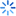• 签到
•苹果/安卓/wp

•苹果/安卓/wp

客户端

• 统计与数据分析
• 经济学
• 管理学
• 金融学
• 财会类
• 国际贸易类
• 考研考博与考证
• 找数据和资料
• 求职与职场
• 学术与投稿类
• 社会生活
• 其它1442875
Date: 07/04/12   Time: 16:48
Trend assumption: Linear deterministic trend
Series: ___02 ____01
Lags interval (in first differences): 1 to 1

Unrestricted Cointegration Rank Test (Trace)

Hypothesized                Trace        0.05
No. of CE(s)        Eigenvalue        Statistic        Critical Value        Prob.**

None         0.458636         14.80961         15.49471         0.0632
At most 1         0.152776         3.150012         3.841466         0.0759

Trace test indicates no cointegration at the 0.05 level
* denotes rejection of the hypothesis at the 0.05 level
**MacKinnon-Haug-Michelis (1999) p-values

Unrestricted Cointegration Rank Test (Maximum Eigenvalue)

Hypothesized                Max-Eigen        0.05
No. of CE(s)        Eigenvalue        Statistic        Critical Value        Prob.**

None         0.458636         11.65960         14.26460         0.1241
At most 1         0.152776         3.150012         3.841466         0.0759

Max-eigenvalue test indicates no cointegration at the 0.05 level
* denotes rejection of the hypothesis at the 0.05 level
**MacKinnon-Haug-Michelis (1999) p-values

Unrestricted Cointegrating Coefficients (normalized by b'*S11*b=I):

___02        ____01
33.10584         241.1798
60.57244         23.18033

D(___02)         0.004018         0.003348
D(____01)        -0.005533         0.001411

1 Cointegrating Equation(s):                 Log likelihood         128.6137

Normalized cointegrating coefficients (standard error in parentheses)
___02        ____01
1.000000         7.285113
(1.69906)

Adjustment coefficients (standard error in parentheses)
D(___02)         0.133033
(0.08218)
D(____01)        -0.183174
(0.05994)haoyun010加载中...